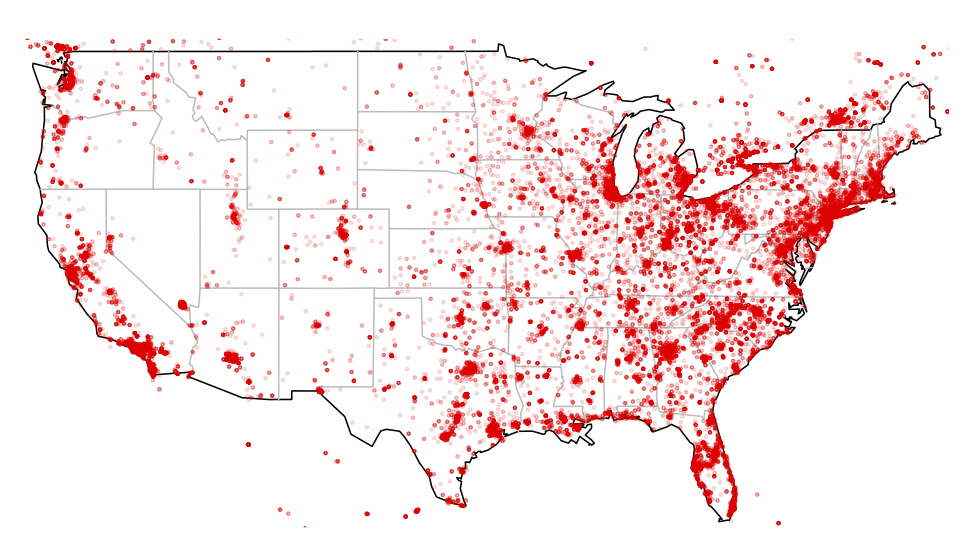# DIY ZeroAccess GeoIP Plots

Since F-Secure was #spiffy enough to provide us with GeoIP data for mapping the scope of the ZeroAccess botnet, I thought that some aspiring infosec data scientists might want to see how to use something besides Google Maps & Google Earth to view the data.

If you look at the CSV file, it’s formatted as such (this is a small portion…the file is ~140K lines):

```CL,"-34.9833","-71.2333" PT,"38.679","-9.1569" US,"42.4163","-70.9969" BR,"-21.8667","-51.8333"```

While that’s useful, we don’t need quotes and a header would be nice (esp for some of the tools I’ll be showing), so a quick cleanup in `vi` gives us:

```Code,Latitude,Longitude CL,-34.9833,-71.2333 PT,38.679,-9.1569 US,42.4163,-70.9969 BR,-21.8667,-51.8333```

With just this information, we can see how much of the United States is covered in ZeroAccess with just a few lines of R:

```# read in the csv file bots = read.csv("ZeroAccessGeoIPs.csv")   # load the maps library library(maps)   # draw the US outline in black and state boundaries in gray map("state", interior = FALSE) map("state", boundary = FALSE, col="gray", add = TRUE)   # plot the latitude & longitudes with a small dot points(x=bots\$Longitude,y=bots\$Latitude,col='red',cex=0.25)```

Can you pwn me now?Click for larger map

If you want to see how bad your state is, it’s just as simple. Using my state (Maine) it’s just a matter of swapping out the `map` statements with more specific data:

```bots = read.csv("ZeroAccessGeoIPs.csv") library(maps)   # draw Maine state boundary in black and counties in gray map("state","maine",interior=FALSE) map("county","maine",boundary=FALSE,col="gray",add=TRUE)   points(x=bots\$Longitude,y=bots\$Latitude,col='red',cex=0.25)```

We’re either really tech/security-savvy or don’t do much computin’ up hereClick for larger map

Because of the way the `maps` library handles geo-plotting, there are points outside the actual map boundaries.

You can even get a quick and dirty geo-heatmap without too much trouble:

```bots = read.csv("ZeroAccessGeoIPs.csv")   # load the ggplot2 library library(ggplot2)   # create an plot object for the heatmap zeroheat <- qplot(xlab="Longitude",ylab="Latitude",main="ZeroAccess Botnet",geom="blank",x=bots\$Longitude,y=bots\$Latitude,data=bots) + stat_bin2d(bins =300,aes(fill = log1p(..count..)))   # display the heatmap zeroheat```Click for larger map

Try playing around with the `bins` to see how that impacts the plots (the `stat_bin2d(…)` divides the “map” into “buckets” (or bins) and that informs plot how to color code the output).

If you were to pre-process the data a bit, or craft some ugly R code, a more tradtional choropleth can easily be created as well. The interesting part about using a non-boundaried plot is that this ZeroAccess network almost defines every continent for us (which is kinda scary).

That’s just a taste of what you can do with just a few, simple lines of R. If I have some time, I’ll toss up some examples in Python as well. Definitely drop a note in the comments if you put together some #spiffy visualizations with the data they provided.Amazon Author Page

## 4 Comments →DIY ZeroAccess GeoIP Plots

1.Jay Jacobs

Here are two changes to your initial elegant and compact version. I first handle the CSV in R so I don’t have to edit the source and then I changed the color to have a low alpha value to allow the points to “stack up”, so areas with more dots appear to be more dense: “red” = “#FF0000” = “#FF0000FF”, I reduce that alpha down with that last bit to “#FF000033”

# creates a character vector of the coordinates

coord.vector <- unlist(strsplit(as.character(bots\$V2), c(",")))

# convert that into a data frame

mapdata <- data.frame(matrix(as.numeric(coord.vector), ncol=2, byrow=T))

# name the rows

colnames(mapdata) <- c("lat", "long")

library(maps)

# draw the US outline in black and state boundaries in gray

map("state", interior = FALSE)
map("state", boundary = FALSE, col="gray", add = TRUE)

# with a low alpha so the color is transparent and can "stack"

points(x=mapdata\$long,y=mapdata\$lat,col='#FF000033',cex=0.25)

1.hrbrmstr

Definitely agree it makes the map that much clearer. For folks who aren’t playing along at home, here’s a sample of @jayjacobs’ modified version:(right-click/save-as for the larger version)

This site uses Akismet to reduce spam. Learn how your comment data is processed.